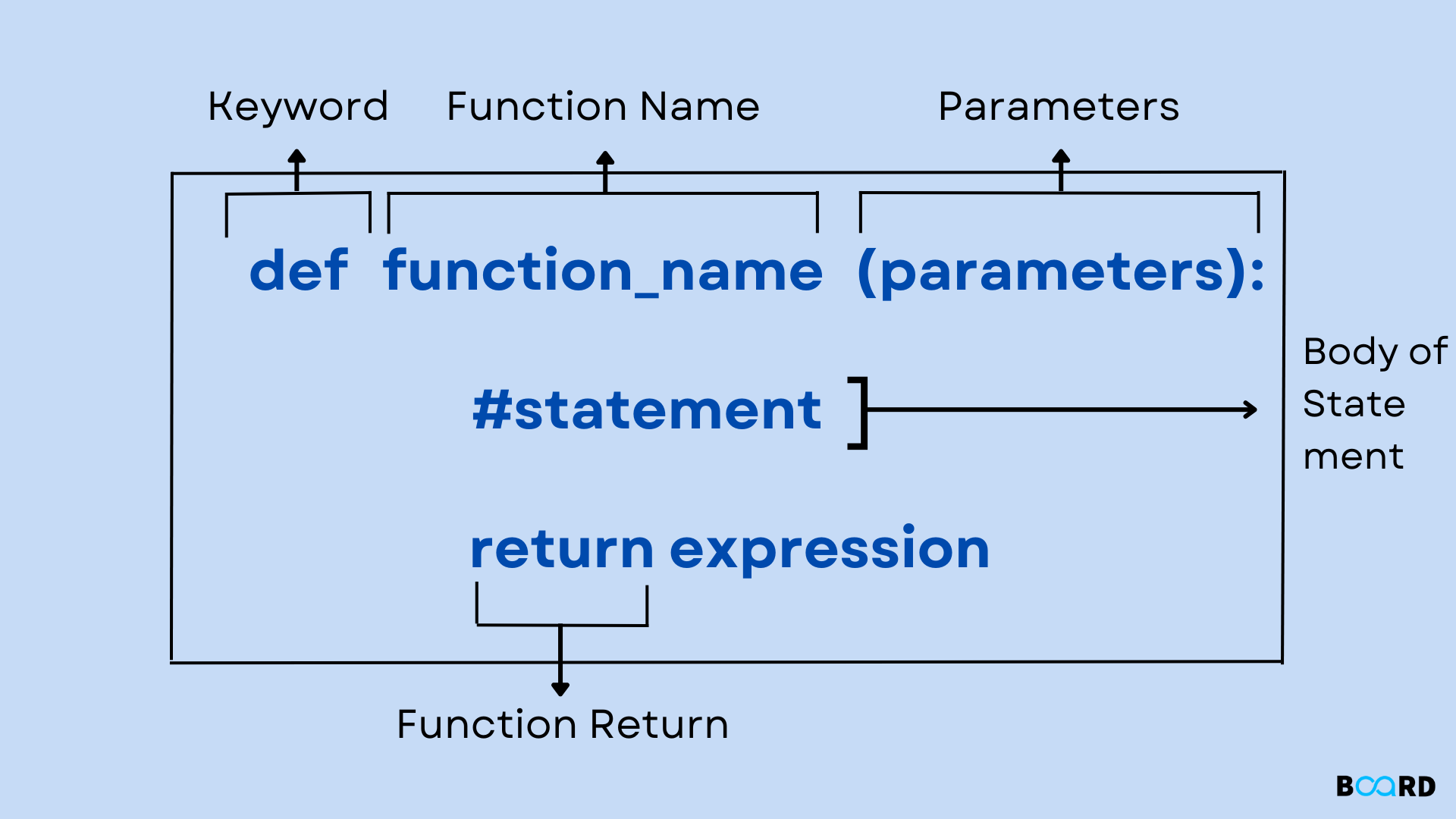# Python Function## Introduction

You'll learn about Python functions and their uses in this article as we write the python programmes in an efficient way. You will gain knowledge beginning with what they are, their syntax, and examples of how to use them in programs.

In Python, a function is a group of linked statements that perform a certain task. By using functions, we may break our software into smaller, more manageable modules. Functions therefore aid us in better structuring and managing our programme as it grows. With the help of functions, we can also get rid of duplication and broaden the scope of our code. Essentially, there are two types of functions:

• User-defined functions – The user defines these functions to carry out a certain task.
• Built-in functions – These Python functions are built-in functions..

## Creating a Function

It includes the def keyword, which is used to define the function in Python. Let's now examine the defined function's syntax.

Syntax:

 def my_function(parameters):            function_blockreturn expression

Let's examine the definition of the syntax of functions:

• We combine the function name and the def keyword to define the function.
• The function name must come after the identifier rule.
• A function will accept an optional parameter or argument.
• A colon (:) is used to indicate the beginning of a function block, and block statements must be indented equally.
• We employ the return statement to return the function's value. There can only be one return statement in a function.

## Function Calling

In Python, a function can be called from another function after it has been created. If a function is not defined before it is called, the Python interpreter will raise an error. When we wish to call a function, we put the function name in parentheses.

### The return statement

The return statement, which is used in functions to help us return the function's result, is used at the end of the function. This statement ends the execution of the function and sends the result to the place where the function was called. The return statement is only usable within the function; take note of this.

Syntax:

 return [expression_list]

It may include an expression that is evaluated and the result is passed back to the calling function. The None object is returned if the return statement contains neither an expression nor an instance of itself within the function.

## Example 1: Adding a Return Statement to a Function

 # Defining functiondef sum():   a = 20   b = 40   c = a+b   return c# calling sum() function in print statementprint("The sum is given by:",sum())

Output:

 The sum is given by: 60
write your code here: Coding Playground

The function sum has been defined in the code above, and it contains the statement c = a+b, which computes the inputted values and returns the result to the calling function.

## Example #2: Writing a function without a return statement

 # Defining functiondef sum():   a = 20   b = 40   c = a+b# calling sum() function in print statementprint(sum())

Output:

 None
write your code here: Coding Playground

The same function is defined in the code above, but it is used without a return statement this time, and we can see that the sum() function returns the None object to the calling function.

## Arguments in function

The function can accept several kinds of data as parameters. In the parenthesis, the arguments are listed. Any number of parameters can be passed to the functions, but each argument must be separated by a comma. Let's examine the example that is provided, which has a function that takes a string as an input.

### Example 1

 # Defining the functiondef func (name):    print("Hi ",name)# Calling the functionfunc("BoardInfinity")

Output:

 Hi BoardInfinity

### Example 2

def sum (num1,num2):
return num1+num2;
num1 = int(input("Enter val: "))
num2 = int(input("Enter val2: "))
print("Sum = ",sum(num1,num2))

Output

 Enter val: 20Enter val2: 40Sum =  60
write your code here: Coding Playground

## Advantages of Functions in Python

• Using functions, we may avoid writing the same logic or code in software many times.
• In a single application, we can call Python functions everywhere and more than once.
• We can easily trace a Python programme that has been divided up into numerous functions.
• The best feature of Python functions is their reusability.
• However, a Python programme always requires more effort when calling functions.